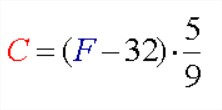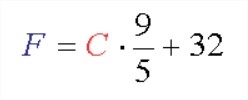# Java Program Convert Fahrenheit To Celsius | Vice Versa

Java Program to convert Fahrenheit to Celsius – Here we discuss the various methods to convert the Fahrenheit temperature measurement to Celsius temperature measurement and vice versa. The various methods include Static Method, Switch Case and the method. We have added the compiler to each case with sample outputs citing specific examples.

The following program has been written in 4 Possible Ways:

• Static Method
• Using Method
• Fahrenheit to Celsius and Vice Versa Using Switch Case
• Celsius To Fahrenheit

Celsius Temperature Scale: Earlier known as the Centigrade Scale, the Celsius Scale is a widely used one, also an SI derived unit for temperature. The normal scale of a Celsius thermometer measures from 0°C (Water’s freezing point at Standard Atmospheric Pressure) to 100°C (Water’s boiling point at Standard Atmospheric Pressure)

Fahrenheit Temperature Scale:  The Fahrenheit Scale is specifically used in the U.S.A and few other places. The normal scale of a Fahrenheit thermometer ranges from 32°F (Water’s freezing point at Std Atm Pressure) and 212°F (Water’s boiling point at Std Atm Pressure)

The formula to convert Fahrenheit into Celsius:The formula to convert Celsius to Fahrenheit:## Using Static Method

1) Read the temperature value using scanner object as sc.nextInt() and store it in the variable a.

In this program, we have the static method celsius(double f), which converts the Fahrenheit temperature into Celsius temperature by using the formula (f-32)*5/9.

2) In the main method, call the celsius method as celsius(a), then celsius(double f) method returns the Celsius temperature.

Output:

### Fahrenheit to Celsius Using method

1) In this program celsius(double f) is the method which calculates the Celsius temperature to the given Fahrenheit temperature using the formula (f-32)*5/9.

2) We will call this method using  FahrenheittoCelsius class object “fah” as fah.celsius(a), this method returns the double data type value.

Output:

#### Fahrenheit to Celsius and Vice Versa Using Switch Case

1) Read the value using scanner class object sc.nextInt(), and store it in the variable ch. Here ch represents the  type of conversion i.e ch=1 represents  Fahrenheit to Celsius,ch=2 represents  Celsius  to Fahrenheit

2) If ch matches with case 1 then that block will be executed, if ch=2 then case 2 blocks will be executed. If we don’t have a break-in case then switch executes all cases.

3) If none of the cases matches with the switch then default block will be executed.

4) In our example ch=1 so case 1: will be executed and calculates the Celsius temperature using the formulas

c=(f-32)*5/9. it has “break” so it comes out of the switch.

if ch=2, then switch search for the case which matches with ch=2, so case 1 doesn’t match so come to case 2 and executes the case 2 block. Here case 1 will not be executed.

Output:

##### C To F using Static Method

1) In this program, we have the static method fahrenheit(double c) which converts the Celsius temperature into Fahrenheit temperature using the formula  ((9*c)/5)+32.

2) The main method which is static calls the static method fahrenheit(double c) as fahrenheit(a) then it returns the Fahrenheit temperature.

Output:

###### C To F using Method

1) double fahrenheit(double c) is the method which calculates fahrenheit temperature to the given celsius temperature using the formula  ((9*c)/5)+32.

2) We will call this method using CelsiustoFahrenheit class object “cel” as cel.fahrenheit(a), then it returns the value, and assigned to the variable “result”.

x

## Plus Star Pattern Java Program | Patterns

Java program to print plus star pattern program – We have written the below print/draw ...